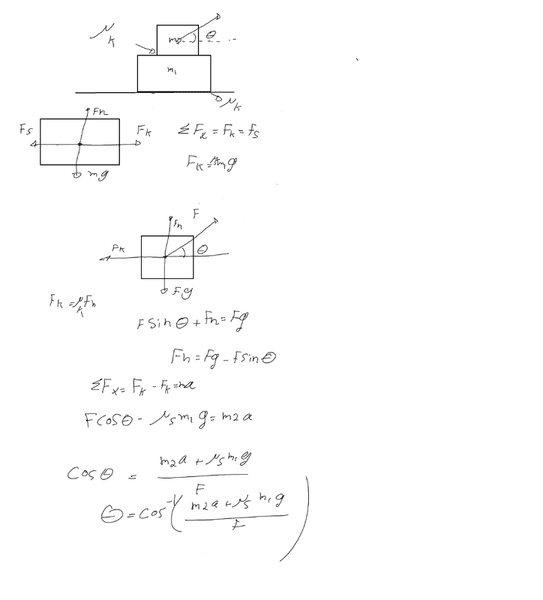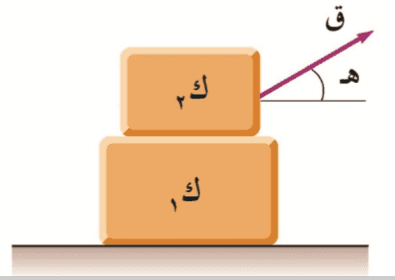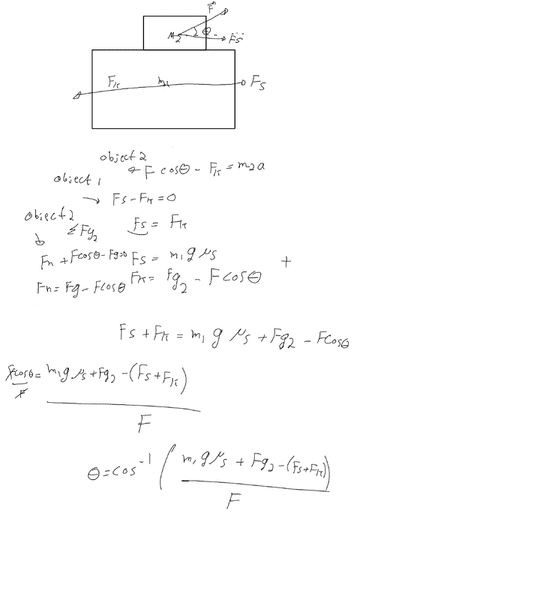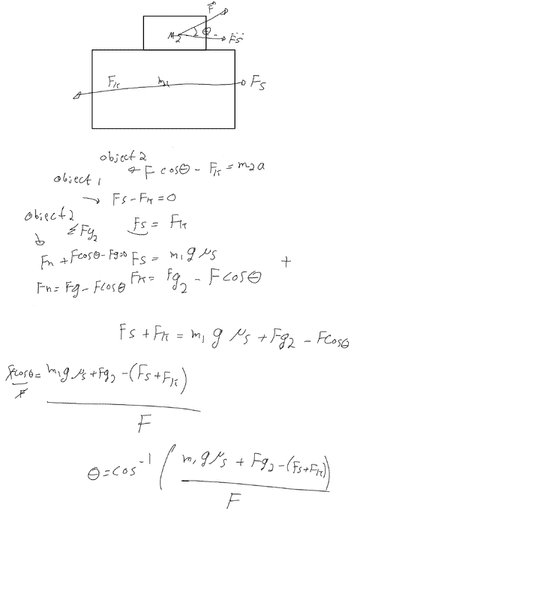# Angle of applied force

• rashida564
In summary, the problem involves finding the minimum angle for a force to be applied in order for box number 1 to remain in static position while box number 2 moves. The scenario includes kinetic and static friction between the boxes and the ground. The problem statement does not provide information about the direction or magnitude of the force, the initial motion of the boxes, or the constraints on the force. The equations of motion for each box must be set up, taking into account the normal and friction forces in both the x and y directions.

## Homework Statement

what is the angle the force should apply in order to be box number 1 in static position and box number 2 move

F=ma

## The Attempt at a Solution•Bana Alkurdi and Delta2
You need to provide a more complete problem statement. Helpers should be able to understand the entirety of the problem by reading the problem statement. You've left out all the details about the scenario, including what friction(s) if any apply and where, whether there are any given values for the various parameters (such as the masses and force), whether the force is directed into the block or is pulling, and so on. New information should not be introduced in the attempt at solution portion of the post.

there is a kinetic friction between box 1 and box 2 and uk and there is a static friction between box 1 and the ground us

box 1 has mass of m1
box 2 has mass of m2

Your information about the scenario is still lacking. What direction is the force applied (pushing into block m2 or pulling block m2)? Do we assume that m1 is already in motion or does it begin at rest? If it starts at rest, does static friction apply initially between the blocks?

What constraints are there on the magnitude of force F? There will likely be a minimum magnitude of force F that can work for some particular angle. For some sufficient force F It is possible that there will be a range of angles that will work. Are you meant to find the minimum force or the range of angles?

If this problem was given as an exercise for a course, I find it hard to believe that it would be so poorly structured. How was this problem supplied to you? Can you provide an image of the problem in its original form?

m1 and m2 start from rest.
they don't give me the magnitude of force.

Sorry, but the original picture was written in Arabic.can anyone help me

I suppose the magnitude of force is given equal to ##F## and we want to find the range of angles ##\theta## (or there might be a unique angle ##\theta##). Also I suppose the whole setup is such that there would be no motion observed in the y-axis.

You have to apply Newton's 2nd law to for each body to make 2 equations for each body:1 for forces on the x-axis and 1 for forces on the y-axis. But be careful these equations can be coupled in various ways since the normal forces that will appear in the y-axis equations, will also appear in the x-axis because they are responsible for the friction forces acting on the x-axis.

You got to be more careful on the forces on the y-axis. I see one of your equation is ##F_n=F_g-F\sin{\theta}## where ##F_g=m_2g## I suppose. This is the normal force that the m2 body applies to the m1 body. So the friction acting on the m2 body in the x-axis should be ##n_kF_n## so the equation for the m2 body in the x-axis should be ##F\cos{\theta}-n_k(m_2g-F\sin{theta})=m_2a_2##. More or less there are the two equations for the m2 body.

Now work your way on making the equations for the m1 body, 1 in x-axis and 1 equation in y-axis.rashida564 said:Since all starts from rest, you only need to get mass 2 to start moving while mass 1 stays still. That means you only need to overcome static friction on the one and not on the other. Kinetic friction does not enter into the equations at all.
Further, the actual acceleration is irrelevant; it can be so small as to be effectively zero,

Your equations are confusing because there are two normal forces but you only have one Fn symbol. When you correct the friction you will have two static friction forces, but they still need distinct labels.

You have an equation Fn+Fcos(θ)-Fg=0. That makes no sense since Fn is vertical and the other two are horizontal.

Posting working as an image makes it hard to make comments. Please take the trouble to type your working in so that others can quote individual lines. Images are for diagrams and textbook extracts.

As Delta2 hinted, your question statement must be incomplete. I assume it asks for the least angle so that block 1 stays still.

•rashida564